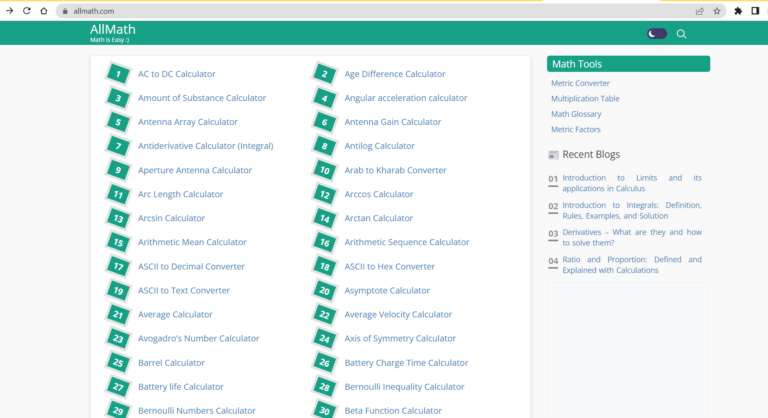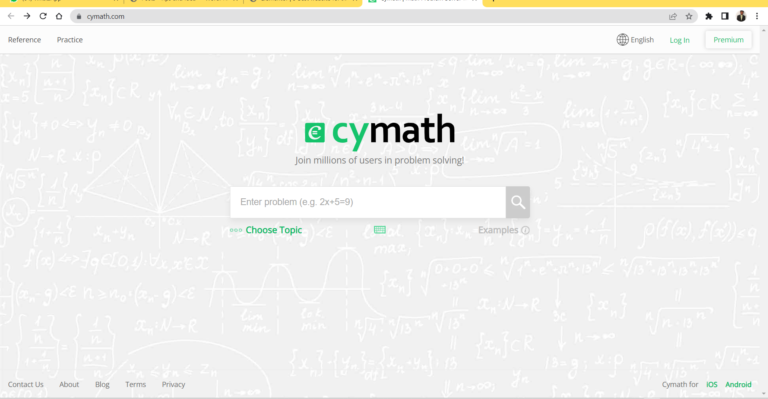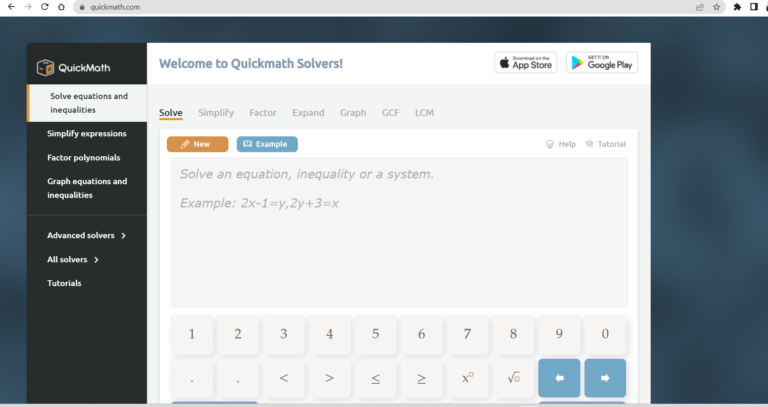### 5 Best website for solve all mathematic problems

Most of the students in school and colleges feel too tough in a subject is Mathematic (Maths). Recently many websites give the best solution for learning mathematics easily. Here below is a list of a few best websites to learn maths online easily.

## Allmath.com

Allmath is one best website for mathematic calculator users. On this website you will get more than 450+  types of calculators online. This website is very user-friendly and it’s free to use. By using this website, students can solve a lot of mathematical and physical solutions.## Cymath.com

Cymath.com is one best website for mathematical questions and answers. By using this website, you can do mathematical solutions like Differentiate, Expand, Factor, Graphs, Integrate, Simplify, and Solve equations. And then Reference and Practice option will help you improve your mathematic skills. This website is free for basic uses and the premium option starts at \$5/month.## QuickMath

QuickMath is one best mathematical-solving website. By using this website you can do mathematical solutions like Simplify Factor Expand Graph GCF LCM. And this website is available in application types Android and iOS.## WebMATH

Webmath is a math help website and it provides answers to math questions and problems, as posted by the user, at any time. Math solutions are generated and displayed instantly, as the user enters their math problem and clicks “solve”. In addition to the answer, Webmath also shows the student how to arrive at the answer.## MathPapa

MathPapa is one of the best website for algebra calculators at home. practice and lesson options very useful to improve mathematical skills. And this site premium starts from \$9.99 per month.